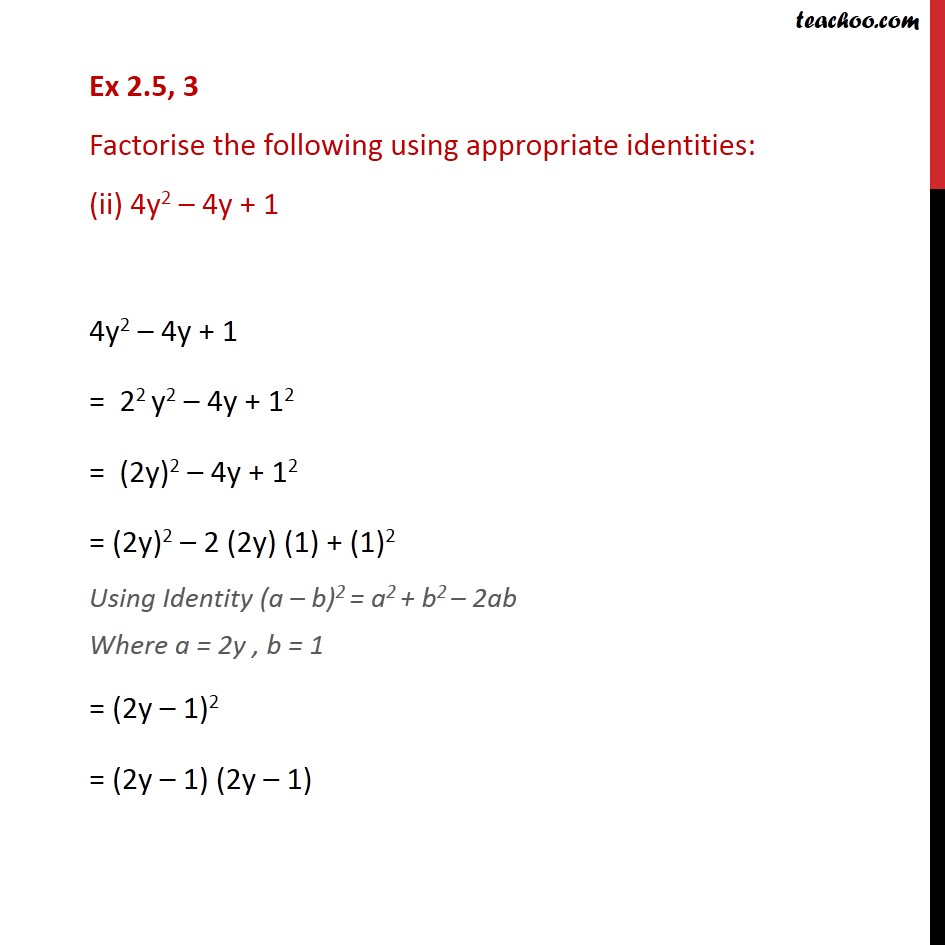Ex 2.4

Chapter 2 Class 9 Polynomials
Serial order wiseLearn in your speed, with individual attention - Teachoo Maths 1-on-1 Class

### Transcript

Ex 2.4, 3 Factorise the following using appropriate identities: (ii) 4y2 4y + 1 4y2 4y + 1 = 22 y2 4y + 12 = (2y)2 4y + 12 = (2y)2 2 (2y) (1) + (1)2 Using Identity (a b)2 = a2 + b2 2ab Where a = 2y , b = 1 = (2y 1)2 = (2y 1) (2y 1)